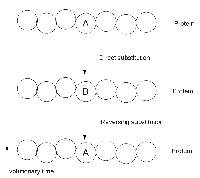# Identifying Reversing Substitutions solved by 123

Dec. 4, 2012, 7:14 a.m. by Naumenko

Topics: Phylogeny

## Reversions Complicate PhylogeniesFigure 1. Illustration of an amino acid's reversing substitution after two point mutations.

In “Fixing an Inconsistent Character Set”, we mentioned how the construction of a phylogeny can be complicated by a the reversion of a character to a past state. For that matter, in calculating Hamming distance and edit distance, the assumption of parsimony required us to assume that if some nucleotide base or amino acid is aligned with an identical symbol in two genetic strings, then it has not changed on the evolutionary path between the two taxa.

However, this model is too strict in practice, where a base or amino acid can change to another state and then change back as the result of two point mutations, which is called a reversing substitution; see Figure 1. In the case of DNA, the presence of only four bases makes randomly occurring reversing substitutions common; these substitutions will carry over into amino acid language via transcription and translation.

Unfortunately, with the possible exception of experimental evolution in bacteria, we lack the luxury of knowing the ancestral state of a nucleic acid strand. Instead, we must infer its most probable ancestor from homologous strands. To do so, we may use characters to construct a phylogeny (see “Character-Based Phylogeny”), then apply alignment-based phylogeny to infer strings for the tree's internal nodes (see “Alignment-Based Phylogeny”). Only once we have an adequate picture of the entire phylogeny, including its internal nodes, can we hope to identify reversing substitutions.

## Problem

For a rooted tree $T$ whose internal nodes are labeled with genetic strings, our goal is to identify reversing substitutions in $T$. Assuming that all the strings of $T$ have the same length, a reversing substitution is defined formally as two parent-child string pairs $(s, t)$ and $(v, w)$ along with a position index $i$, where:

• there is a path in $T$ from $s$ down to $w$;
• $s[i] = w[i] \neq v[i] = t[i]$; and
• if $u$ is on the path connecting $t$ to $v$, then $t[i] = u[i]$.

In other words, the third condition demands that a reversing substitution must be contiguous: no other substitutions can appear between the initial and reversing substitution.

Given: A rooted binary tree $T$ with labeled nodes in Newick format, followed by a collection of at most 100 DNA strings in FASTA format whose labels correspond to the labels of $T$. We will assume that the DNA strings have the same length, which does not exceed 400 bp).

Return: A list of all reversing substitutions in $T$ (in any order), with each substitution encoded by the following three items:

• the name of the species in which the symbol is first changed, followed by the name of the species in which it changes back to its original state
• the position in the string at which the reversing substitution occurs; and
• the reversing substitution in the form original_symbol->substituted_symbol->reverted_symbol.

## Sample Dataset

(((ostrich,cat)rat,mouse)dog,elephant)robot;
>robot
AATTG
>dog
GGGCA
>mouse
AAGAC
>rat
GTTGT
>cat
GAGGC
>ostrich
GTGTC
>elephant
AATTC


## Sample Output

dog mouse 1 A->G->A
dog mouse 2 A->G->A
rat ostrich 3 G->T->G
rat cat 3 G->T->G
dog rat 3 T->G->T